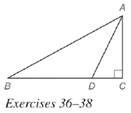Chapter 5.6, Problem 37EElementary Geometry For College St...

7th Edition
Alexander + 2 others
ISBN: 9781337614085

Solutions

Chapter
SectionElementary Geometry For College St...

7th Edition
Alexander + 2 others
ISBN: 9781337614085
Textbook Problem

In right Δ A D C , D C = 5 and A C = 5 3 . If A D → bisects ∠ B A C , find B D .To determine

To find:

BD.

Explanation

Given:

In a right ΔABC with right C, AD bisects BAC and DC=5,AC=53.

Theorem used:

Angle bisector theorem:

If a ray bisects one angle of a triangle, then it divides the opposite side into segments whose lengths are proportional to the lengths of the two sides that form the bisected angle.

Calculation:

Consider a right ΔABC.

Given that AD bisects BAC.

By angle bisector theorem, we get

ABAC=BDDC

ACDC=ABBD

We have DC=5,AC=53.

535=ABBD31=ABBD

AB:BD=3:1

Let AB=3x,BD=x

From the figure we see that, BC=BD+DC

BC=x+5

Consider the right triangle ABC with right angle at C

Still sussing out bartleby?

Check out a sample textbook solution.

See a sample solution

The Solution to Your Study Problems

Bartleby provides explanations to thousands of textbook problems written by our experts, many with advanced degrees!

Get Started

Convert the expressions in Exercises 8596 radical form. 34/5

Finite Mathematics and Applied Calculus (MindTap Course List)

In Problems 23-28, graph the functions.

Mathematical Applications for the Management, Life, and Social Sciences

For

Study Guide for Stewart's Multivariable Calculus, 8th

Sometimes, Always, or Never: limxaf(x)g(x)=limxaf(x)limxag(x).

Study Guide for Stewart's Single Variable Calculus: Early Transcendentals, 8th